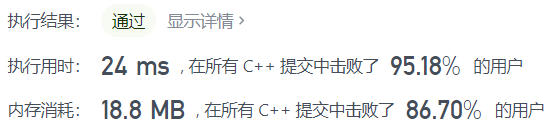# leedcode 49. 字母异位词分组（哈希，字符串内排序）

## 示例

1 <= strs.length <= 104
0 <= strs[i].length <= 100
strs[i] 仅包含小写字母

## 题解

• 哈希 字符串内字符排序
• 思路方法都能很快想到，代码也能熟练打出来，就是对 vector<vector<string>>ans ，map 使用不够熟练。
• 同一组的字符串，对其内字符排序后都是相同的，用它作为 哈希 的键值以示区分。
• 字符串内排序  sort(astr.begin(),astr.end());
class Solution {
public:
vector<vector<string>> groupAnagrams(vector<string>& strs) {
unordered_map<string,int>str_map;//哈希 排序后的字符串与整数一一对应
vector<vector<string>>ans;//存放结果
int n=strs.size();
int count=1;//哈希对应的整数值 不从0开始 因为如果没有对应的字符串返回也是0 以区分
for(int i=0;i<n;i++){
string stmp=strs[i];

string new_stmp = stmp;//对字符串内字符排序
sort(new_stmp.begin(),new_stmp.end());

if(str_map[new_stmp]){//如果哈希表中有这个字符串
int index=str_map[new_stmp]-1;//那么找到对应的整数
ans[index].push_back(stmp);//在对应的vector中放入 原字符串
}else{//如果没有出现过
str_map[new_stmp]=count;//那就新设置一个映射整数值
vector<string>vtmp;//新建一个vector
vtmp.push_back(stmp);//在新建一个vector中放入 原字符串
ans.push_back(vtmp);//再将这个新的vector放入结果中
count++;//映射整数值记得要更新
}
}
return ans;
}
};• 官方 也是 哈希 排序
• 代码非常简洁，它的哈希是  unordered_map<string, vector<string>> mp; 字符串与一个 vector 进行映射，并且 vector  是会变化，运行中会增加元素的。
• 由于 unordered_map<string, vector<string>> mp，所以在运行 mp[key].emplace_back(str) 时，如果 mp[key] 还没有 vector<string>，emplace_back 会新建一个 vector<string>，在这个新建的 vector 中放入str，再把这整个 vector 与key对应,也就是一个字符串与整个vector对应。如果 mp[key] 已经有 vector<string>，就会把 str 直接放入已有的 vector 中。也可以用 push_back。
class Solution {
public:
vector<vector<string>> groupAnagrams(vector<string>& strs) {
unordered_map<string, vector<string>> mp;
for (string& str: strs) {//遍历strs中的每个字符串
string key = str;
sort(key.begin(), key.end());//对字符串内的字符排序
mp[key].emplace_back(str);//新建一个vector,放入str,再与key对应,也就是一个字符串与整个vector对应,用 push_back 是一样的
}
vector<vector<string>> ans;
for (auto it = mp.begin(); it != mp.end(); it++) {//遍历哈希表
ans.emplace_back(it->second);//用 push_back 是一样的
}
return ans;
}
};


tips：如何清除 vector 中的数据

        // 如何清除vector中的数据""
vector<string>::iterator it = strs.begin();
while(it != strs.end()){
if(*it==""){
strs.erase(it);
}
else it++;
}
posted on 2021-09-19 23:37  蔡军帅  阅读(37)  评论(0编辑  收藏  举报